# Signal and Image Noise Models¶

Important: Please read the installation page for details about how to install the toolboxes. $\newcommand{\dotp}{\langle #1, #2 \rangle}$ $\newcommand{\enscond}{\lbrace #1, #2 \rbrace}$ $\newcommand{\pd}{ \frac{ \partial #1}{\partial #2} }$ $\newcommand{\umin}{\underset{#1}{\min}\;}$ $\newcommand{\umax}{\underset{#1}{\max}\;}$ $\newcommand{\umin}{\underset{#1}{\min}\;}$ $\newcommand{\uargmin}{\underset{#1}{argmin}\;}$ $\newcommand{\norm}{\|#1\|}$ $\newcommand{\abs}{\left|#1\right|}$ $\newcommand{\choice}{ \left\{ \begin{array}{l} #1 \end{array} \right. }$ $\newcommand{\pa}{\left(#1\right)}$ $\newcommand{\diag}{{diag}\left( #1 \right)}$ $\newcommand{\qandq}{\quad\text{and}\quad}$ $\newcommand{\qwhereq}{\quad\text{where}\quad}$ $\newcommand{\qifq}{ \quad \text{if} \quad }$ $\newcommand{\qarrq}{ \quad \Longrightarrow \quad }$ $\newcommand{\ZZ}{\mathbb{Z}}$ $\newcommand{\CC}{\mathbb{C}}$ $\newcommand{\RR}{\mathbb{R}}$ $\newcommand{\EE}{\mathbb{E}}$ $\newcommand{\Zz}{\mathcal{Z}}$ $\newcommand{\Ww}{\mathcal{W}}$ $\newcommand{\Vv}{\mathcal{V}}$ $\newcommand{\Nn}{\mathcal{N}}$ $\newcommand{\NN}{\mathcal{N}}$ $\newcommand{\Hh}{\mathcal{H}}$ $\newcommand{\Bb}{\mathcal{B}}$ $\newcommand{\Ee}{\mathcal{E}}$ $\newcommand{\Cc}{\mathcal{C}}$ $\newcommand{\Gg}{\mathcal{G}}$ $\newcommand{\Ss}{\mathcal{S}}$ $\newcommand{\Pp}{\mathcal{P}}$ $\newcommand{\Ff}{\mathcal{F}}$ $\newcommand{\Xx}{\mathcal{X}}$ $\newcommand{\Mm}{\mathcal{M}}$ $\newcommand{\Ii}{\mathcal{I}}$ $\newcommand{\Dd}{\mathcal{D}}$ $\newcommand{\Ll}{\mathcal{L}}$ $\newcommand{\Tt}{\mathcal{T}}$ $\newcommand{\si}{\sigma}$ $\newcommand{\al}{\alpha}$ $\newcommand{\la}{\lambda}$ $\newcommand{\ga}{\gamma}$ $\newcommand{\Ga}{\Gamma}$ $\newcommand{\La}{\Lambda}$ $\newcommand{\si}{\sigma}$ $\newcommand{\Si}{\Sigma}$ $\newcommand{\be}{\beta}$ $\newcommand{\de}{\delta}$ $\newcommand{\De}{\Delta}$ $\newcommand{\phi}{\varphi}$ $\newcommand{\th}{\theta}$ $\newcommand{\om}{\omega}$ $\newcommand{\Om}{\Omega}$

This numerical tour show several models for signal and image noise. It shows how to estimate the noise level for a Gaussian additive noise on a natural image. It also shows the relevance of thresholding to remove Gaussian noise contaminating sparse data.

In :
warning off
warning on


## Additive Gaussian Noise Model ¶

The simplest noise model consist in adding a realization of a zero mean random vector to a clean signal or image.

Load a clean image.

In :
N = 128;
name = 'boat';
M0 = rescale(crop(M0,N));


Load a clean signal.

In :
n = 1024;
name = 'piece-regular';
f0 = rescale( load_signal(name,n) );


The simplest noise model is Gaussian white noise. Here we generate a noisy signal or image.

In :
sigma = .1;
M = M0 + randn(N,N)*sigma;
f = f0 + randn(n,1)*sigma;


Display the signals.

In :
clf;
subplot(3,1,1);
plot(f0); axis([1 n 0 1]);
title('Clean signal');
subplot(3,1,2);
plot(f-f0); axis([1 n -3*sigma 3*sigma]);
title('Noise');
subplot(3,1,3);
plot(f); axis([1 n 0 1]);
title('Noisy signal');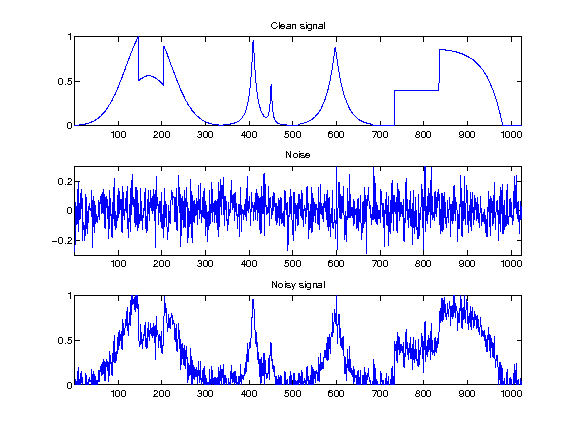Display the images.

In :
clf;
imageplot(M0, 'Clean image', 1,3,1);
imageplot(M-M0, 'Noise', 1,3,2);
imageplot(clamp(M), 'Noisy image', 1,3,3);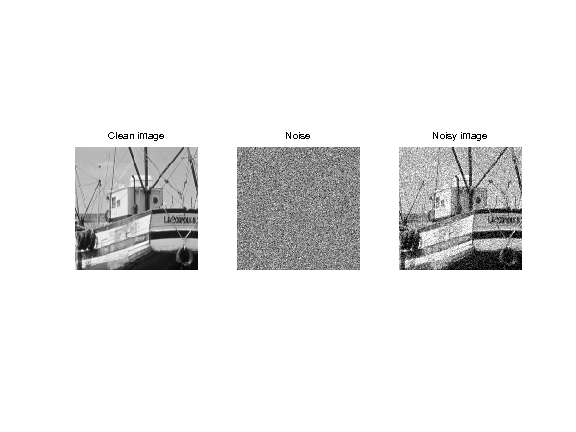Display the statistics of the noise

In :
nbins = 51;
[h,t] = hist( M(:)-M0(:), nbins ); h = h/sum(h);
subplot(3,1,2);
bar(t,h);
axis([-sigma*5 sigma*5 0 max(h)*1.01]);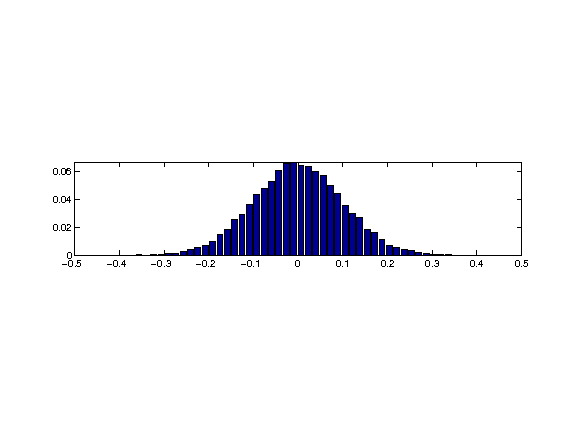## Additive Uniform Noise¶

A slightly different kind of noise is uniform in a given interval.

Generate noisy data with uniform noise distribution in |[-a,a]|, with |a| chosen so that the variance is sigma.

In :
a = sqrt(3)*sigma;
M = M0 + 2*(rand(N,N)-.5)*a;
f = f0 + 2*(rand(n,1)-.5)*a;


Display the signals.

In :
clf;
subplot(3,1,1);
plot(f0); axis([1 n 0 1]);
title('Clean signal');
subplot(3,1,2);
plot(f-f0); axis([1 n -3*sigma 3*sigma]);
title('Noise');
subplot(3,1,3);
plot(f); axis([1 n 0 1]);
title('Noisy signal');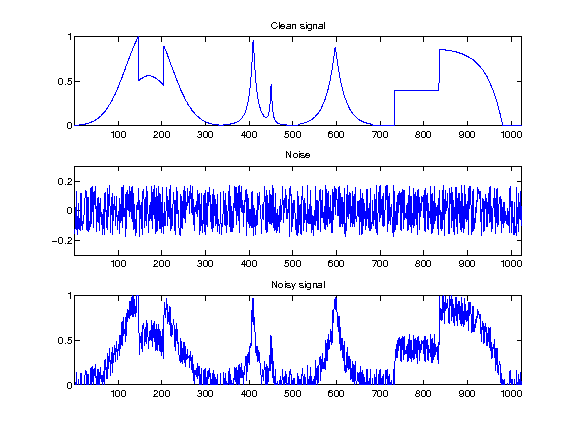Display the images.

In :
clf;
imageplot(M0, 'Clean image', 1,3,1);
imageplot(M-M0, 'Noise', 1,3,2);
imageplot(clamp(M), 'Noisy image', 1,3,3);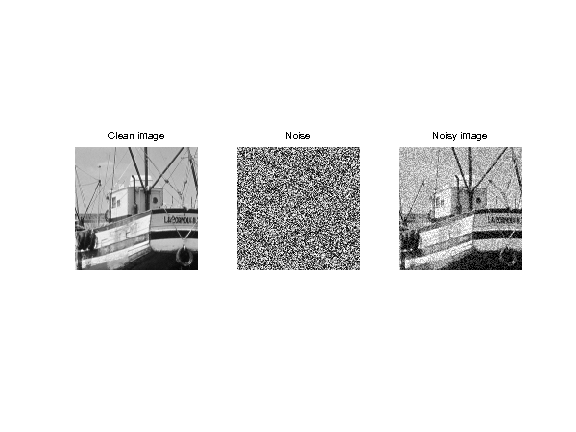Display the statistics of the noise

In :
nbins = 51;
[h,t] = hist( M(:)-M0(:), nbins ); h = h/sum(h);
subplot(3,1,2);
bar(t,h);
axis([-sigma*5 sigma*5 0 max(h)*1.01]);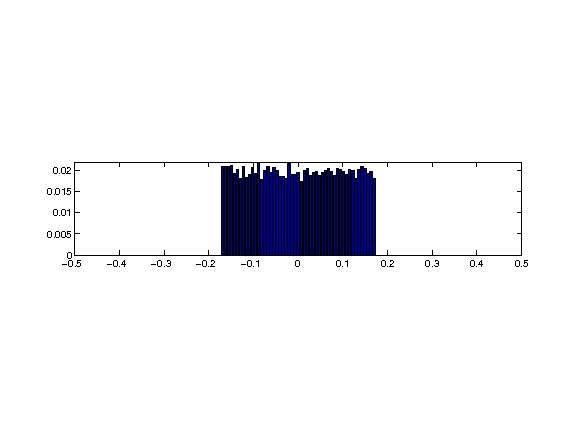## Impulse Noise¶

A very different noise model consist in sparse impulsions, generate by a random distribution with slowly decaying probability.

Generate noisy image with exponential distribution, with variance |sigma|.

In :
W = log(rand(N,N)).*sign(randn(N,N));
W = W/std(W(:))*sigma;
M = M0 + W;


Generate noisy signal with exponential distribution, with variance |sigma|.

In :
W = log(rand(n,1)).*sign(randn(n,1));
W = W/std(W(:))*sigma;
f = f0 + W;


Display the signals.

In :
clf;
subplot(3,1,1);
plot(f0); axis([1 n 0 1]);
title('Clean signal');
subplot(3,1,2);
plot(f-f0); axis([1 n -3*sigma 3*sigma]);
title('Noise');
subplot(3,1,3);
plot(f); axis([1 n 0 1]);
title('Noisy signal');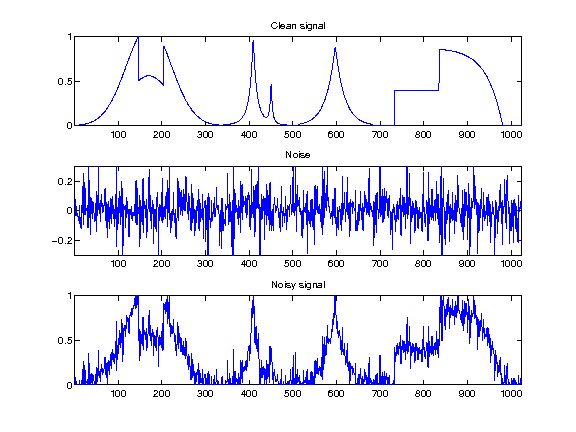Display the images.

In :
clf;
imageplot(M0, 'Clean image', 1,3,1);
imageplot(M-M0, 'Noise', 1,3,2);
imageplot(clamp(M), 'Noisy image', 1,3,3);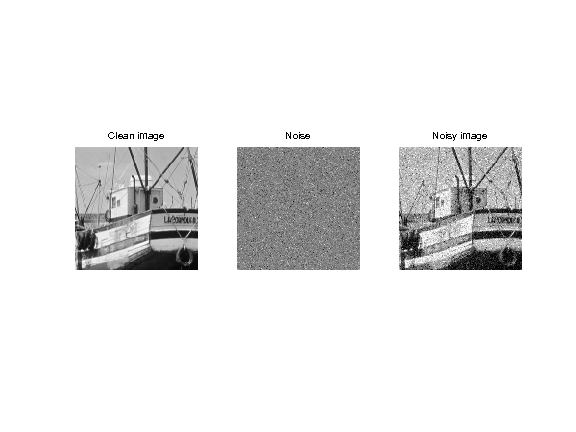Display the statistics of the noise

In :
nbins = 51;
[h,t] = hist( M(:)-M0(:), nbins ); h = h/sum(h);
subplot(3,1,2);
bar(t,h);
axis([-sigma*5 sigma*5 0 max(h)*1.01]);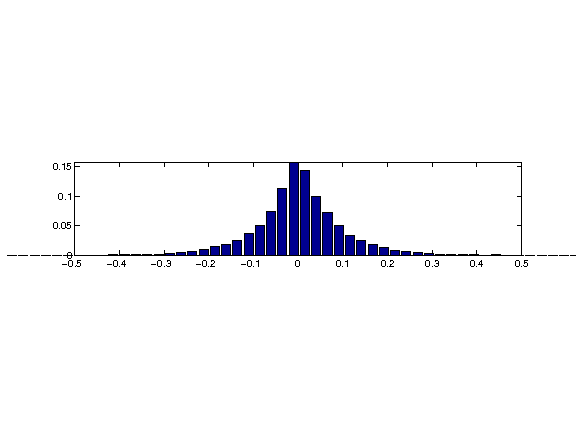## Thresholding Estimator and Sparsity¶

The idea of non-linear denoising is to use an orthogonal basis in which the coefficients |x| of the signal or image |M0| is sparse (a few large coefficients). In this case, the noisy coefficients |x| of the noisy data |M| (perturbated with Gaussian noise) are |x0+noise| where |noise| is Gaussian. A thresholding set to 0 the noise coefficients that are below |T|. The threshold level |T| should be chosen judiciously to be just above the noise level.

First we generate a spiky signal.

dimension

In :
n = 4096;


probability of spiking

In :
rho = .05;


location of the spike

In :
x0 = rand(n,1)<rho;


random amplitude in [-1 1]

In :
x0 = 2 * x0 .* ( rand(n,1)-.5 );


We add some gaussian noise

In :
sigma = .1;
x = x0 + randn(size(x0))*sigma;


Display.

In :
clf;
subplot(2,1,1);
plot(x0); axis([1 n -1 1]);
set_graphic_sizes([], 20);
title('Original signal');
subplot(2,1,2);
plot(x); axis([1 n -1 1]);
set_graphic_sizes([], 20);
title('Noisy signal');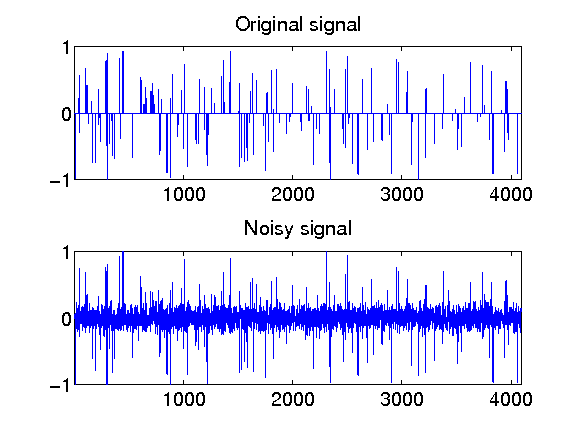Exercise 1

What is the optimal threshold |T| to remove as much as possible of noise ? Try several values of |T|.

In :
exo1()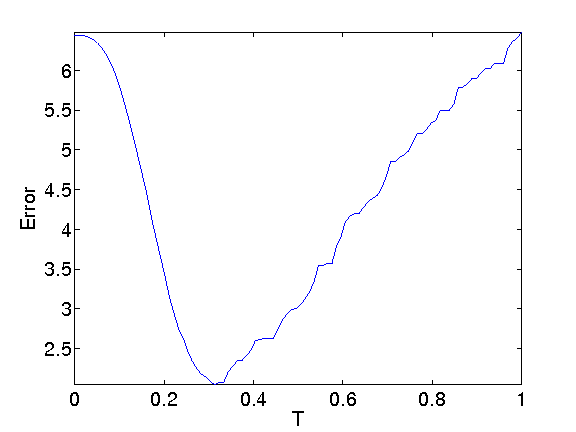In :
%% Insert your code here.


In order to be optimal without knowing in advance the amplitude of the coefficients of |x0|, one needs to set |T| just above the noise level. This means that |T| should be roughly equal to the maximum value of a Gaussian white noise of size |n|.

Exercise 2

The theory predicts that the maximum of |n| Gaussian variable of variance |sigma^2| is smaller than |sqrt(2*log(n))| with large probability (that tends to 1 when |n| increases). This is also a sharp result. Check this numerically by computing with Monte Carlo sampling the maximum with |n| increasing (in power of 2). Check also the deviation of the maximum when you perform several trial with |n| fixed.

In :
exo2()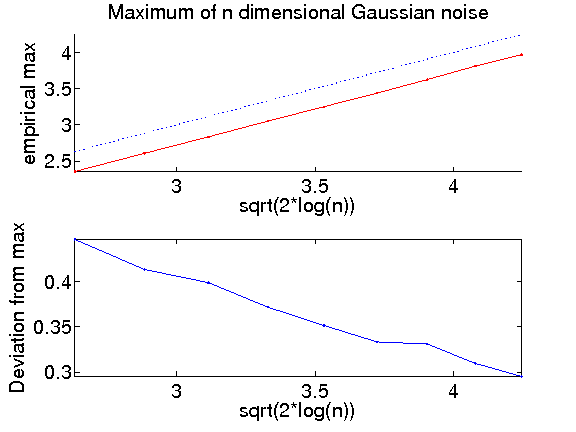In :
%% Insert your code here.


## Estimating the noise level¶

In practice, the noise level |sigma| is unknown. For additive Gaussian noise, a good estimator is given by the median of the wavelet coefficients at the finer scale. An even simple estimator is given by the normalized derivate along X or Y direction

Load a clean image.

In :
n = 256;
M0 = rescale(load_image('boat', n));


Generate a noisy image.

In :
sigma = 0.06;
M = M0 + randn(n,n)*sigma;


First we extract the high frequency residual.

In :
H = M;
H = (H(1:n-1,:) - H(2:n,:))'/sqrt(2);
H = (H(1:n-1,:) - H(2:n,:))'/sqrt(2);


Display.

In :
clf;
imageplot(clamp(M), 'Noisy image', 1,2,1);
imageplot(H, 'Derivative image', 1,2,2);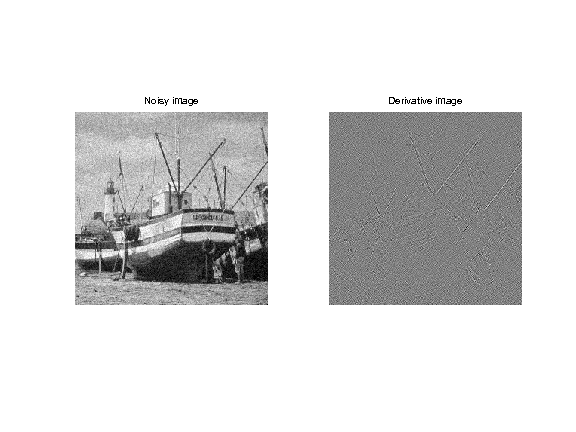Histograms.

In :
[h,t] = hist(H(:), 100);
h = h/sum(h);


Display histogram.

In :
clf;
bar(t, h);
axis([-.5 .5 0 max(h)]);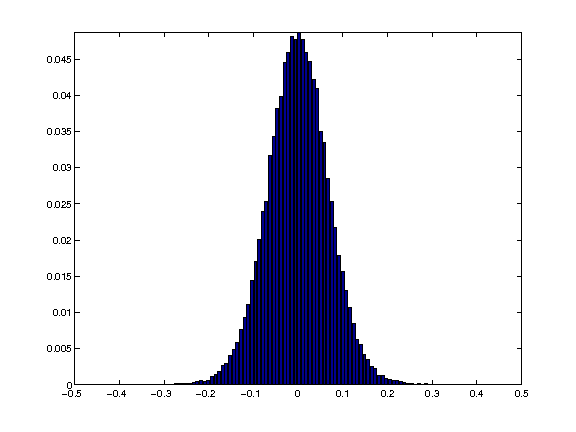The mad estimator (median of median) must be rescaled so that it gives the correct variance for gaussian noise.

In :
sigma_est = mad(H(:),1)/0.6745;
disp( strcat(['Estimated noise level=' num2str(sigma_est), ', true=' num2str(sigma)]) );

Estimated noise level=0.066828, true=0.06